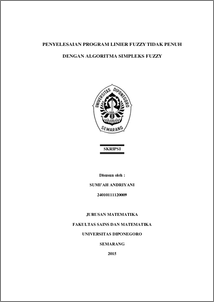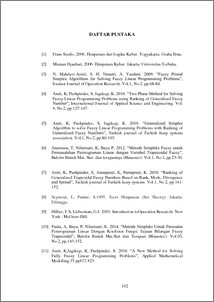# PENYELESAIAN PROGRAM LINIER FUZZY TIDAK PENUH DENGAN ALGORITMA SIMPLEKS FUZZY

ANDRIYANI, SUMI’AH (2015) PENYELESAIAN PROGRAM LINIER FUZZY TIDAK PENUH DENGAN ALGORITMA SIMPLEKS FUZZY. Undergraduate thesis, UNDIP.Preview
PDF
685KbPDF
Restricted to Repository staff only

2571KbPreview
PDF
190Kb

## Abstract

A generalized simplex algorithm is proposed for solving a special type of fuzzy linear programming (FLP) problems. This generalized simplex algorithm belong to extention from classical simplex algorithm. To determine Entering variable (EV) or Leaving variable (LV) in simplex table can be used rank function. This final task discuss about simplex algorithm to finish not full FLP with number of trapezoidal generalized fuzzy. Standart form trapezoidal generalized fuzzy that is (a,b,c,d;w) where 𝑤 ∈ [0,1]. A generalized simplex algorithm can be used to get optimal fuzzy solution from not full fuzzy linear program problems with unequal constraint. Fuzzy optimal solution obtained from the simplex algorithm is the same value on trapezoidal fuzzy numbers for any chosen of value 𝑤. Keywords : Fuzzy linear programming, trapezoidal generalized fuzzy, simplex algorithm

Item Type: Thesis (Undergraduate) Q Science > QA Mathematics Faculty of Science and Mathematics > Department of Mathematics 84301 INVALID USER 14 Jun 2022 10:04 14 Jun 2022 10:04

Repository Staff Only: item control page